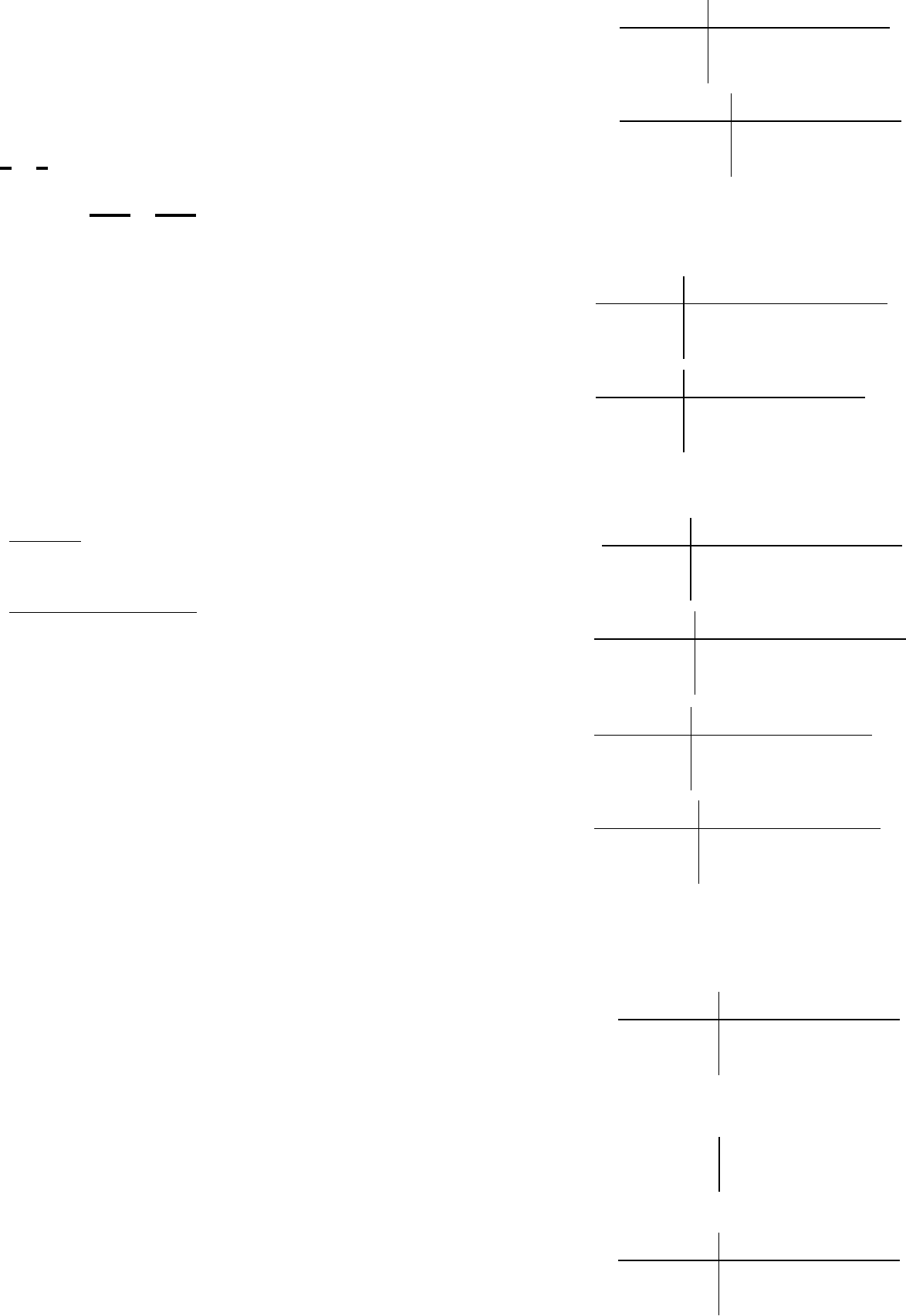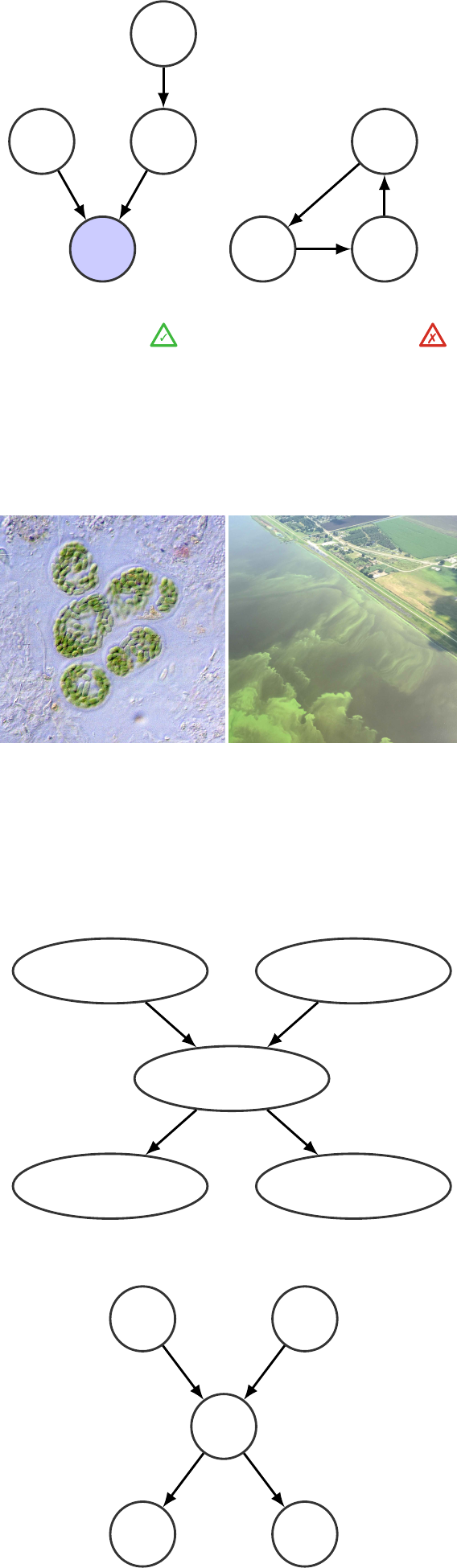11
Bayesian Networks
Bayesian networks were introduced by Pearl
1
and are also known
1
Pearl, J. (1988). Probabilistic reasoning in
intelligent systems: Networks of plausible
inference.MorganKaufmann
as belief networks and directed graphical models. They are t h e result
of the combination of probabili ty theory covered in chapter 3 with
graph theory, which employs graphs deﬁned by links and nodes.
We saw in
§
3.2 that the chain rule allows formulating the joint
probability for a set of random variables using conditional and
marginal probabilities, for example,
p
(
x
1
,x
2
,x
3
)=
p
(
x
3
|x
2
,x
1
)
p
(
x
2
|x
1
)
p
(
x
1
).
Bayesian networks (BNs) employ nodes to represent random vari-
ables and directed links to describe the dependencies between them.
Bayesian networks are probabilistic models where the goal is to
learn the joint probability deﬁned over the e ntire network. The joint
probability for the structure encoded by these nodes and links is
formulated using the chain rule. The key with Bayesian networks is
that they allow building sparse models for which ecient variable
elimination algorithms exist in order to estimate any conditional
probabilities from the j oi nt probability.
BNs can be categorized as unsupervised l earning
2
where the goal
2
Ghahramani, Z. (2004). Unsupervised
learning. In Advanced lectures on ma-
chine learning,Volume3176,pp.72112.
Springer
is to estimate the joint probability density function (PDF) for a set
of observed variable s. In it s most gener al form we may seek to learn
the structure of the BN itself. In this chapter, we restrict ourselves
to the case where we know the graph structure, and the goal is to
learn to predict unobserved quantities given some observed ones.
T
V
F
Temperature: t 2{cold, hot}
Virus: v 2{yes, no}
Flu: f 2{sick, ¬sick}
Figure 11.1: Example of Bayesian network
for representing the relationships b etween
temperature, the presence of the ﬂu v irus,
and being sick from the ﬂu virus.
Flu vi ru s example Section 3.3.5 presented the distinction between
correlati on and causality using the ﬂu virus example. A Bayesian
network can be employed to model the dependence between the
temperatu re, the presence of the ﬂu virus, and being sick from the
ﬂu. We model our knowledge of t he se three quantities using discrete
random variables that are represented by the nodes in ﬁgure 11.1.
The arrows represent the dependencies between variab l es : The
temperature
T
aects the virus prevalence
V
, which in turns aectsj.-a. goulet 168
the probability of catching the vir us an d being sick
F
. The absence
T
and
F
indicates that the temperature and
being sick from the ﬂu are conditionally independen t from each
other. In the context of this example, conditional independence
implies that
T
and
F
are independent when
V
is known. The joint
probability for T , V , and F ,
p(f,v,t) =
=p(f|v,t)
z }| {
p(f|v) ·p(v|t) · p(t)
| {z }
p(v,t)
,
is obtained using the chain rule where, for each arrow, the cond i-
tional probabilities are described in a conditional probability table.
Virus example: Marginal and condi-
tional probability tables
p(t) = {p(cold),p(hot)} = {0.4, 0.6}
p(v|t) =
8
<
:
t =cold t =hot
v =yes 0.80.1
v =no 0.20.9
p(f|v) =
8
<
:
v =yes v =no
f =sick 0.70
f = ¬sick 0.31
Joint probability using chain rule
p(v, t) = p(v|t) · p(t)
=
8
<
:
t =cold t =hot
v =yes 0.8 0.40.1 0.6
v =no 0.2 0.40.9 0.6
=
8
<
:
t =cold t =hot
v =yes 0.32 0.06
v =no 0.08 0.54
p(f, v,t) = p(f |v) · p(v, t)
=
8
>
>
>
>
>
>
>
<
>
>
>
>
>
>
>
:
f =sick t =cold t =hot
v =yes 0.320.70.060.7
v =no 0.08 00.54 0
f = ¬sick t =cold t =hot
v =yes 0.320.30.060.3
v =no 0.08 10.54 1
=
8
>
>
>
>
>
>
>
<
>
>
>
>
>
>
>
:
f =sick t =cold t =hot
v =yes 0.224 0.042
v =no 00
f = ¬sick t =cold t =hot
v =yes 0.096 0.018
v =no 0.08 0.54
Variable elimination: Marginalization
p(f, t) =
X
v
p(f, v,t)
=
8
<
:
t =cold t =hot
f =sick 0.224 0.042
f = ¬sick 0.176 0.558
p(f) =
X
t
p(f, t)
=
f =sick 0.266
f = ¬sick 0.734
p(t|f) = p(f, t)/p(f)
=
8
<
:
t =cold t =hot
f =sick 0.84 0.16
f = ¬sick 0.24 0.76
In the case where we observe
F
=
f
, we can employ the
marginalization operation in order to obtain a conditional prob-
ability quantifying how the observation
f 2{sick, ¬sick}
changes
the probability for the tempe rat u re T ,
p(t|f) =
p(f,t)
p(f)
=
P
v
p(f,v,t)
P
t
P
v
p(f,v,t)
.
In minimalistic problems such as this one, it is trivial to calculate
the joint probability using the chain rule and eliminating variables
using marginalization. However, in practical cases involving dozens
of variables with as many links between them, these calcul at i ons
become computationally demanding. Moreover, in practice, we
seldom know the marginal and conditional probabilities tables.
A key interest of working with directed graphs is that ecient
estimation methods are available to perform all t h ose tasks.
Bayesian networks are applicable not only for discrete ran-
dom variables but also for continuous ones, or a mix of both. In
this chapter, we restrict ourselves to the study of BN for discrete
random variables. Note that the state-space models presented in
chapter 12 can be seen as a time-dependent Bayesian network using
Gaussian random variables with linear dependence models. This
chapter presents the nomenclature employed to deﬁne graphi c al
models, the methods for per for mi n g infe re nc e, and the methods
allowing us to learn the conditional probabilities deﬁning the de-
pendencies between random variables. In addition, we present an
introduction to time-dependent Bayesian networks that are referred
to as dynamic Bayesi an networks. For advanced topics regarding
Bayesian networks, the reader is invited to consult specialized text-
book s such as the one by Nielsen and Jensen
3
or Murphy’s PhD
3
Nielsen, T. D. and F. V. Jensen (2007).
Bayesian networks and decision graphs.
Springer
thesis.
4
4
Murphy, K. P. (2002). Dynamic Bayesian
networks: representation, inference and
learning.PhDthesis,UniversityofCalifor-
nia, Berkeleyprobabilistic machine learning for civil engineers 169
11.1 Graphical Models Nomenclature
Bayesian networks employ a special type of graph: directed acyclic
graph (DAG). A DAG
G
=
{U, E}
is deﬁned by a set of node s
U
interconnected by a set of directed links
E
. In order to be acycl i c,
the directed links between variables cannot be deﬁned such th at
there are self-loops or cycles in the graph. For a set of random vari-
ables, there are many ways to deﬁne links between variables, each
one leading to the same joint probability. Note that dir ect e d link s
between variables are not required to describe causal relationships.
Despite causality not being a requirement, it is a key to ecien cy ;
if the directed links in a graphical model are assigned following the
causal relationships, it generally produces sparse models requiring
the deﬁnition of a smaller number of conditional probabilities than
noncausal counterparts.
X
2
X
3
X
1
X
4
(a) Directed acyclic
graph (DAG)
X
2
X
3
X
1
(b) Directed graph
containing a cycle
Figure 11.2: Example of dependence
hidden (white) and an observed (shaded)
nodes describing random variables.
Figure 11.2a presents a directed acyclic graph and ( b) p r es ents a
directed graph containing a cycle so that it cannot be modeled as a
Bayesian network. In ﬁgure 11.2a random variables are represented
by nodes, where the observed variable
X
4
depends on the hidden
variables
X
2
and
X
3
. The directions of links indicate that
X
2
and
X
3
are the parent of
X
4
, that is,
parents
(
X
4
)=
{X
2
,X
3
}
, and
consequently,
X
4
is the child of
X
2
and
X
3
. Each child is asso c iat e d
with a conditional probability table (CPT) whose size depends on
the number of parents,
p
(
x
i
|parents
(
X
i
)). Nodes without parents
are described by their marginal prior probabilities
p
(
x
i
). The joint
PDF
p
(
U
) for the entire Bayesian network is formulated using the
chain rule,
p(U)=p(x
1
,x
2
, ··· ,x
X
)=
X
Y
i=1
p(x
i
|parents(X
i
)).
This application of the chain rule requires that given its parents,
each node is independent of its other ancestors.
(a) Cyanobacteria
seen under a micro-
scope
(b) Cyanobacteria
bloom in a rural
environment
Figure 11.3: Example of cyanobacteria
bloom. (Photo: NASA and USGS)
Cyanobacteria
Fish mortality
Water color
Temperature
Fertilizer
(a) Bayesian network semantic representa-
tion
C
M W
T F
(b) Bayesian network represented with
random variables
Figure 11.4: Bayesian network for the
cyanobacteria example.
Cyanobacteria example We explore the example illustrated in
ﬁgure 11.3 of cyanobacteria blooms that can o cc ur in lakes, rivers,
and estuaries. Cyanobacteria blooms are typically caused by the
use of fertilizers that wash into a water body, combined with warm
temperatures that allow for bacteria reproduction. Cyanobacteria
blooms can cause a change in the water color and can cause ﬁsh or
marine life mortality. We employ a Bayesian network to des cr i be
the joint probability for a set of random variables consisting of the
temperature
T
, the use of fertilizer
F
, the presence of cyanobacteria
in water
C
, ﬁsh mortality
M
, and the water color
W
. In ﬁgure 11.4,